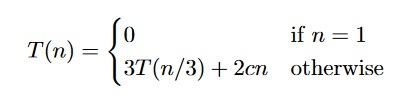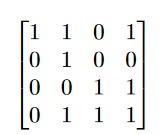# COMP90038 Algorithms and Complexity

```{`
The University of Melbourne
School of Computing and Information Systems
COMP90038 Algorithms and Complexity
Assignment 1, Semester 1 2020
`}```

## Objectives

To improve your understanding of the time complexity of algorithms and recurrence relations. To develop problem-solving and design skills. To improve written communication skills; in particular the ability to present algorithms clearly, precisely and unambiguously.

### Problems

[4 marks] Consider the following pseudocode:
x ← 0
for k ← 1 to n do
for j k to 2n do
x x + 1

• How many times is the inner-most statement (x x+1 ) executed? Justify your answer using the summation notation and rules as described in Appendix 1 of Levitin.
• Express the time complexity of the code segment using the appropriate Big O//Θ That is, you should make the strongest possible claim about the complexity of the code segment.
1. [4 marks] Consider the following recurrence relation:where c is a positive constant. Assume that n = 3m.

Solve the recurrence. Your answer should show how you derive the closed form.

1. [8 marks] A manager wants to purchase two different products from a list of n products with a fixed budget k. Assume the prices of the n products are distinct, which are stored in an array A sorted in increasing order of price.

Design an algorithm to find how many different combinations that the manager can choose from. For example, if there are five different products, of which the prices are A = [1,2,3,4,6] the budget k = 4, the algorithm should return 2 as only the combinations of (1,2) and (1,3) do not exceed the budget.

Note, that the algorithm would be incorrect if it returned a value of 1 corresponding to the product in A = 4 as this would not meet the criteria of ‘two different products’ given the budget constraint.

Full marks will be given if your algorithm runs in time. Your algorithm should be presented in unambiguous pseudocode or Python code.

1. [4 marks] Your colleague has partially implemented an algorithm to test if a strongly connected graph is bipartite (can be 2-coloured). Pseudocode for her algorithm is presented below.

Your colleague has won the lottery and decided to leave university, so it is up to you to finish the algorithm. Unfortunately, she did not define the data structure for the variable X. However, you can tell from the documentation that the data structure was supposed to be either a stack or a queue.

function IsBipartite(G[0...n − 1][0...n − 1]) . Input is an adjacency matrix n × n

numSeen = 0

colour[0...n-1] ← [-1, ···, -1]

X ← EmptyQueueOrStack( ) PushOrEnqueue(X, <0, RED>) while numSeen < n do i, c ← PopOrDequeue(X) if colour[i] = -1 then

numSeen ← numSeen + 1

colour[i] ← c

nextColour ← RED if c = RED then nextColour ← BLUE

for j ← 0 to n − 1 do if G[i][j] = 1 then if colour[j] = c then return false

PushOrEnqueue(X, <j, nextColour>)

return true

• Will a stack data structure or queue data structure produce the correct answer?
• Provide an adjacency matrix for a small graph that causes the other data structure to either return the wrong result or not terminate. (Hint: a 3 node graph is sufficient)
1. [10 marks] You are an employee of The Department of Environments and Fire Assessment. You have been assigned a task to write an algorithm to calculate the size of the largest connected burnt area in the state of Victoria as a result of the devastating recent bushfires.

• We will represent the environment using a 2D array (or an abstract map of the area under consideration). Here, the actual scale is not relevant in the problem formulation.
• Each cell in the 2D array contains a binary variable indicating whether the cell has been burnt or not. For example the value of cell ci,j = 1 if the area represented by that site has been burnt. In contrast, the value of cell ci,j = 0 if the area represented by that site has not been burnt
• A ‘connected burnt area’ is defined as a collection of connected cells where ci,j = 1 (ie., burnt) for all cells. Two cells cm,n and cx,y are connected if either of the cells is a north, south, east, or west neighbour of the other.

For example, in the 2D array below, the size of the largest connected burnt area is 5.Your algorithm should be presented in unambiguous pseudocode or Python code. Note: it may be necessary to modularise your solution using multiple functions.

Full marks will only be awarded if your algorithm is correct with appropriate time complexity. Here, we have not provided a clear definition of ‘appropriate time complexity’. We do this, as we want you to think carefully about the design of your solution – lots and lots of nested for loops is not the correct approach to use.

Hints:

• For each cell ci,j, determine which of the other cells it is connect to.
• How could a graph traversal algorithm be used in this context?